Next: Preliminary properties Up: LOCALLY CONNECTED CONTINUA Previous: Graphs

Dendrites

A dendrite is a locally connected continuum containing no simple closed curve. By the order of a point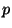in a dendrite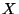, writing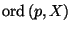, we mean the Menger-Urysohn order, see [Kuratowski 1968, §51, I, p. 274], or equivalently, the order in the classical sense, i.e., the number of arcs emanating fromand disjoint out of(see [Charatonik 1962, p. 229] and [Lelek 1961, p. 301]).

In this chapter the term of an end point of a continuum is always used in the sense of a point of (Menger-Urysohn) order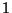, i.e.,is an end point ofprovided that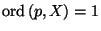. For dendrites this concept coincides with one of an end point in the classical sense, but not with the notion of an end point of an arc-like continuum as defined e.g. in [Bing 1951, p. 660].

Given a dendrite, we denote by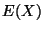the set of all end points ofand by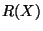the set of all its ramification points (i.e., points of order at least 3). Various structural as well as mapping characterizations of dendrites are collected in [Charatonik et al. 1998, Theorems 1.1 and 1.2, p. 228 and 230, respectively]. See also [Charatonik et al. 2000].

SubsectionsNext: Preliminary properties Up: LOCALLY CONNECTED CONTINUA Previous: Graphs
Janusz J. Charatonik, Pawel Krupski and Pavel Pyrih
2001-11-30